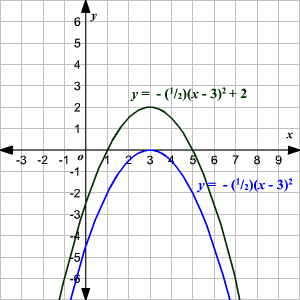# Graphing Quadratic Equations Using Transformations

A quadratic equation is a polynomial equation of degree $2$ .  The standard form of a quadratic equation is

$0=a{x}^{2}+bx+c$

where   $a,b$ and $c$ are all real numbers and $a\ne 0$ .

If we replace $0$ with $y$ , then we get a quadratic function

$y=a{x}^{2}+bx+c$

whose graph will be a parabola .

Sometimes by looking at a quadratic function, you can see how it has been transformed from the simple function $y={x}^{2}$ . Then you can graph the equation by transforming the "parent graph" accordingly.  For example, for a positive number $c$ , the graph of $y={x}^{2}+c$ is same as graph $y={x}^{2}$ shifted $c$ units up. Similarly, the graph $y=a{x}^{2}$ stretches the graph vertically by a factor of $a$ . (Negative values of $a$ turn the parabola upside down.)

We can see some other transformations in the following examples.

Example 1:

Graph the function $y=2{x}^{2}-5$ .

If we start with $y={x}^{2}$ and multiply the right side by $2$ , it stretches the graph vertically by a factor of $2$ .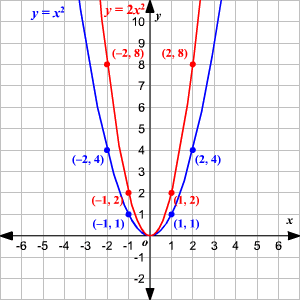Then if we subtract $5$ from the right side of the equation, it shifts the graph down $5$ units.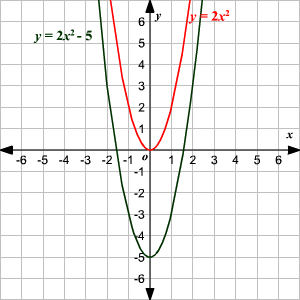Example 2:

Graph the function $y=-\frac{1}{2}{\left(x-3\right)}^{2}+2$ .

If we start with $y={x}^{2}$ and replace $x$ with $x-3$ , it has the effect of shifting the graph $3$ units to the right.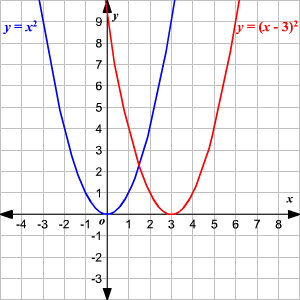Then if we multiply the right side by $-\frac{1}{2}$ , it turns the parabola upside down and gives it a vertical compression (or "squish") by a factor of $2$ .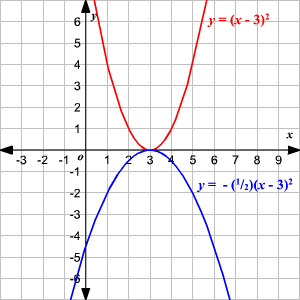Finally, if we add $2$ to the right side, it shifts the graph $2$ units up.